# Geometry

## Geometry: from shapes to transformations

Geometry is a highly visual subject. Our geometry resources begin at the kindergarten level with recognizing simple shapes such as triangles, circles and rectangles and proceed through grade 5 level geometry, including coordinate geometry and analysis of 3-D shapes.

## Geometry topics for kindergarten to grade 5

• identify classify 2-D shapes including triangles, quadrilaterals and circles
• properties of circles
• properties and classification of angles and triangles
• understand spatial relationships (in and out, above and below, etc.)
• subdivide and combine shapes into new shapes
• decompose 2D shapes to develop concept of fractions
• understand the concepts of perimeter and area
• understand congruence, similarity and symmetry
• points, segments, rays and lines
• recognize parallel and perpendicular lines
• coordinate geometry (e.g. find locations on a grid; the four quadrants)
• predict the results of sliding, flipping, and turning two-dimensional shapes (transformations)
• identify 3-D shapes
• surface area and volumes of rectangular prisms
• identify lines of symmetry and rotational symmetry in 2D shapes.
• build 3D objects from 2D representations
• draw 2D representations of 3D objects.

## Geometry worksheets

Our geometry worksheets are organized by grade level:

Kindergarten shapes worksheets

• Tracing, drawing and naming the basic shapes
• Drawing shapes in a pattern
• Is the shape 2D or 3D?

• Matching similar shapes
• Matching shapes to their names
• Identifying and labeling 2-D shapes
• Drawing and identifying squares, rectangles, triangles, circles, ovals, diamonds
• Rotating and scaling 2D shapes

• Identifying 2D shapes including pentagons, hexagons
• Edges and vertices
• Composing and decomposing 2D shapes
• Identifying parts of a whole using shapes
• Congruent shapes
• Lines of symmetry
• Area of a rectangle on a rectangular grid
• Area and perimeter of 2D shapes
• Identifying 3D shapes and matching them to real objects
• Counting edges, faces and vertices

• Parallelograms
• Classifying triangles by their angles (acute / obtuse / right)
• Classifying triangles by their sides (scalene, isosceles, equilateral)
• Properties of circles
• Regular polygons and properties of polygons
• Lines, segments and rays
• Parallel and perpendicular lines
• Classifying and measuring angles
• Drawing angles with a protractor

• Classifying angles
• Classifying triangles
• Perimeter and area of rectangles and irregular rectangular shapes
• Circumference of a circle

• Classify and measure anglesShapes and Geometry Focuses on identifying and describing 2D shapes.  Also position words, rotating and scaling shapes and some 3D shapes.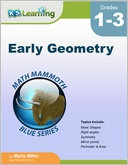Early Geometry Basic shapes (rectangles, squares,...), right angles, symmetry, mirror points, perimeter and area.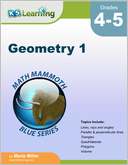Geometry 1 Overview focused on basic plane geometry. Topics include lines, rays and angles; measuring angles; parallel and perpendicular lines, shapes, area, volume. Also rectangular prisms and surface area.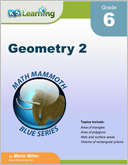Geometry 2 Areas and volumes of common shapes; polygons in a coordinate grid and areas of polygons. Area and perimeter problems, nets, surface area and volume problems including volume of rectangular prisms.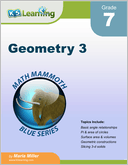Geometry 3 Angles and triangles, geometric constructions, pi and circles, area and perimeter problems, surface area, converting units of area, slicing 3-dimensional shapes and the volume of prisms and cylinders.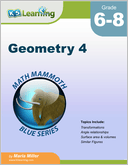Geometric transformations and congruence; translations, reflections, rotations, sequences of transformations. Similar figures, corresponding angles, angle relationships and parallel lines. Volume of prisms, cylinders, pyramids, cones and spheres.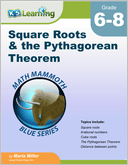Square Roots & the Pythagorean Theorem Square roots, the Pythagorean Theorem, applications of the Pythagorean Theorem and a proof of the Pythagorean Theorem.